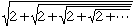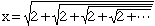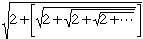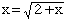Name: Cynthia Who is asking: Parent Level: All Question: justify algebreically, that: 2=the square root of 2 + the square root of 2 + the square root of 2 + the square root of 2 + the square root of 2 + and so on, ....... Hi Cynthia, If you are willing to agree thatis a number then give it a name, say x, soNotice that the part of the following expression in square brackets is a copy of xso,Square both sides and solve for x. Cheers, Penny Go to Math Central